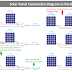In this article, we are going to learn how to connect Solar Panels in Parallel. We will learn what happens if we connect multiple solar panels in parallel if they have the same ratings. Also, we will learn what happens if we connect multiple solar panels in parallel if they have different ratings(means voltage, current, and power).

In Solar power generating systems or Solar Installation for domestic purposes such as in our home, sometimes we need to connect multiple solar panels in parallel. For example, Solar panels have a certain power or current rating at which they operate optimally. By connecting panels in parallel, you can increase the overall system current and power to match the requirements of a higher-powered inverter or battery bank.

There are also some advantages of connecting solar panels in parallel such as, When panels are connected in parallel, the voltage remains the same as that of an individual panel. Lower voltage can reduce the cost of insulation, and ensure electrical safety in wiring and connections. Parallel connections increase the current output of the system. This can be advantageous when working with devices or components that require higher current levels. If you're designing a system for lower voltage applications, parallel connections allow you to achieve the desired current levels while maintaining a manageable system voltage.

## Solar Panel Connection in Parallel with Same Ratings

When we connect multiple solar panels having the same ratings in parallel,
1. The total output voltage will be the same as the voltage output of the individual solar panel.
2. The total output current will be the total or summation of the output current of individual solar panels.
3. The total output power will be the total or summation of the output power of individual solar panels. That is the multiplication of Output Voltage and Output Current.

For Example, If we connect 2 Nos 12V, 50W, 4.17A solar panels in parallel the Total Output will be 12V, 100W, 8.34A

Similarly, If we connect 3 Nos 12V, 50W, 4.17A solar panels in parallel the Total Output will be 12V, 150W, 12.51A.

## Solar Panel Connection in Parallel with Different Ratings

When we connect multiple solar panels having different ratings in parallel,
1. The total output voltage will be equal to the voltage output of the lowest voltage-rated solar panel.
2. The total output current will be the total or summation of the output current of individual solar panels.
3. The total output power will be the multiplication of the Output Voltage and Output Current but it will not be equal to the total or summation of the output power of individual solar panels. Even you will get the low output power.

For Example, If we connect a 12V, 40W, 3.33A solar panel with a 24V, 50W, 2.08A solar panel in parallel the Total Output will be 12V, 65W, 5.41A.

You can see here we get the output power of 65 Watt Only. In actuality, it should be 40W + 50W = 90W.

So don't connect solar panels in parallel if they have different ratings. You will not get the maximum power and the power loss will happen.

**But Remember that you can connect multiple solar panels in parallel having different ratings to get the maximum power output only when the voltage ratings of both solar panels are the same.**

For example, If we connect a 12V, 75W, 6.25A solar panel with a 12V, 50W, 4.17A solar panel in parallel the Total Output will be 12V, 125W, 10.42A.

In this case, you can see, we got the maximum power which is the total or summation of the output power of individual solar panels 75W + 50W = 125W.

So, the conclusion is when connecting multiple solar panels in parallel the voltage ratings of all the panels should be the same. Different current and power ratings can be acceptable. But it is always recommended to connect multiple solar panels having the same ratings.

## How to Calculate the Number of Solar Panels in Parallel?

If you want to calculate how many solar panels you should connect in parallel then follow this formula,

N = Iout/Isolar

Where N = Number of solar panels required

Iout = Output current you required

Isolar = Current ratings of individual solar panels

Generally, we don't calculate the current. We calculate the power that we required. For example, we may need 500W power output with 12V voltage.

And you have 12V, 50W panels.

So in this case you need 500/50 = 10 Nos solar panels that are to be connected in parallel to get the 500W power with 12V voltage.

Thank you for visiting the Website. Keep visiting for more Updates.

Solar Panel Connection Diagram and Wiring in ParallelReviewed by Author on August 16, 2023 Rating: 5